# AP Physics C: Mechanics : Understanding Vector and Scalar Quantities

## Example Questions

### Example Question #1 : Mechanics Exam

Which of the following is not a vector quantity?

Force

Acceleration

Velocity

Speed

Speed

Explanation:

Speed is defined as the magnitude of velocity. In other words, speed visually represents the size of a velocity vector, but is indepedent of its direction.

Velocity, acceleration, and force are all vector quantities. Each is defined by the magnitude of the measurement as well as its direction of action.

### Example Question #2 : Mechanics Exam

A dog is initially standing near a fire hydrant. The dog moves 3 meters to the right. Then it runs 7 meters to the left. What is the dog's final displacement from its original position?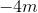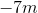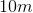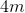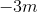Explanation:

This question tests your understanding of displacement as a vector quantity. The best way to solve it is to create a number line to track the motion of the dog.

Let the initial position of the dog be 0m on our number line:Then the dog moves three meters to the right, which we can represent as follows: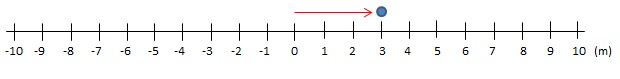Then the dog moves seven meters to the left. Note that it is moving seven meters to the left from the position it is at now (3m):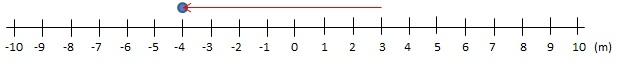We find that at the end of its motion, the dog's displacement is four meters to the left of its original position, which we write as.

Remember that displacement is the change in position, and not the total distance traveled. We only care about the initial position and the final position, regardless of the path traveled. Positive and negative signs indicate the direction of motion.

### Example Question #3 : Mechanics Exam

You drive your car from your house all the way to your school which is 50km away. After you are done with classes you drive back through the same route and park exactly where you had your car at the beginning of the day. By the end of the day, what were the distance and displacement of your motion?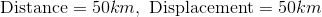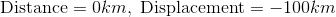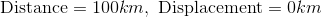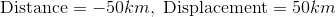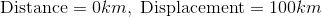Explanation:

This question tests your conceptual understanding of distance as a scalar quantity vs your understanding of displacement as a vector quantity.

Distance measures the total length that was traveled in a given motion, and does not care about the direction since it is a scalar value. In your day, you traveled 50km on your way to school and 50km on your way back home. In total you traveled 100km, so that is your distance.

Displacement is a vector quantity that measures the change in position. It cares about your final and initial positions, taking into account the direction of the change in position. In this scenario you started and ended your motion exactly at the same position, so overall at the end of the day your car did not change position at all. Therefore your displacement was 0m.

### Example Question #4 : Mechanics Exam

An object travels North along a straight line at a constant rate of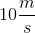. What are the object's speed and velocity?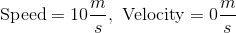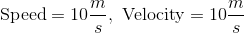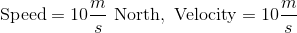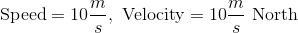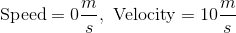Therefore, your speed isand your velocity is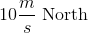.